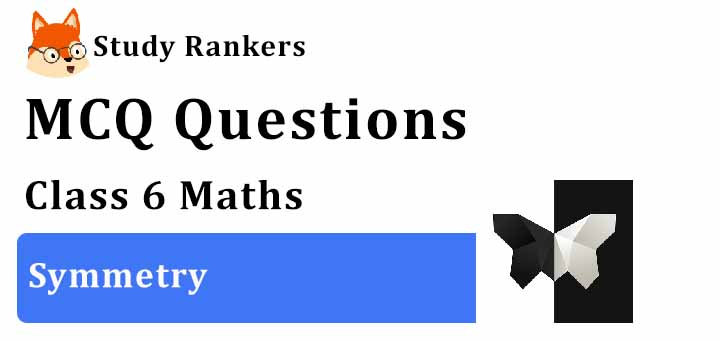>

## MCQ Questions for Class 6 Maths: Ch 13 Symmetry1. Letter ‘H’ of the English alphabet have reflectional symmetry (i.e., symmetry related to mirror reflection) about.
(a) Neither horizontal nor veritcal
(b) Both horizontal nor veritcal
(c) a horizontal mirror
(d) a vertical mirror
► (b) Both horizontal nor veritcal

2. Which of the following alphabets has no line of symmetry?
(a) A
(b) B
(c) Q
(d) O
► (c) Q

3. How many lines of symmetries are there in a square?
(a) 1
(b) 2
(c) 4
(d) 3
► (c) 4

4. In a Δ ABC, AB = AC and AD⊥BC, BE⊥ AC and CF ⊥ AB. Then about which of the following is the triangle symmetrical?
(b) BE
(c) CF
(d) AC

5. How many lines of symmetries are there in rectangle?
(a) 4
(b) 0
(c) 1
(d) None of these
► (d) None of these

6. Which of the following alphabets has many lines of symmetry?
(a) A
(b) O
(c) Q
(d) B
► (b) O

7. Letter ‘E’ of the English alphabet have reflectional symmetry (i.e., symmetry related to mirror reflection) about.
(a) a horizontal mirror
(b) a vertical mirror
(c) both
(d) None of these
► (a) a horizontal mirror

8. Which of the following has 5 lines of symmetry?
(a) A circle.
(b) A regular pentagon.
(c) A triangle.
► (b) A regular pentagon.

9. How many lines of symmetry does a circle have?
(a) One
(b) Two
(c) Three
(d) Many
► (d) Many

10. A rhombus is symmetrical about
(a) the line joining the midpoints of its adjacent sides.
(b) each of its diagonals.
(c) perpendicular bisector of each of its sides.
(d) its sides.
► (b) each of its diagonals.

11. A parallelogram has ______ lines of symmetry:
(a) 0
(b) 1
(c) 2
(d) 3
► (a) 0

12. How many lines of symmetries are there in an isosceles triangle?
(a) 2
(b) 1
(c) 3
(d) None of these
► (b) 1

13. Letter ‘M’ of the English alphabet have reflectional symmetry (i.e., symmetry related to mirror reflection) about.
(a) a vertical mirror
(b) a horizontal mirror
(c) both
(d) None of these
► (a) a vertical mirror

14. The mirror image of ‘W’, when the mirror is placed vertically:
(a) U
(b) M
(c) V
(d) W
► (d) W

15. Which of the following letters of the English alphabet has a vertical line of symmetry?
(a) F
(b) T
(c) E
(d) G
► (b) T

16. Letter ‘G’ of the English alphabet have reflectional symmetry (i.e., symmetry related to mirror reflection) about.
(a) a horizontal mirrorboth
(b) a vertical mirror
(c) both
(d) Neither horizontal nor veritcal
► (d) Neither horizontal nor veritcal

17. Letter ‘D’ of the English alphabet have reflectional symmetry (i.e., symmetry related to mirror reflection) about.
(a) a vertical mirror
(b) both
(c) a horizontal mirror
(d) None of these
► (c) a horizontal mirror

18. How many lines of symmetry does a rectangle have?
(a) One
(b) Two
(c) Three
(d) Many
► (b) Two

19. Which of the following letters have reflection line of symmetry about vertical mirror?
(a) C
(b) B
(c) V
(d) Q
► (c) V

20. How many lines of symmetries are there in a rhombus?
(a) 1
(b) 4
(c) 3
(d) 2
► (d) 2

21. The order of the rotational symmetry of the parallelogram about the centre is
(a) 1
(b) 0
(c) 3
(d) 2
► (b) 0

22. Letter ‘B’ of the English alphabet have reflectional symmetry (i.e., symmetry related to mirror reflection) about.
(a) a horizontal mirror
(b) a vertical mirror
(c) both
(d) None of these
► (a) a horizontal mirror